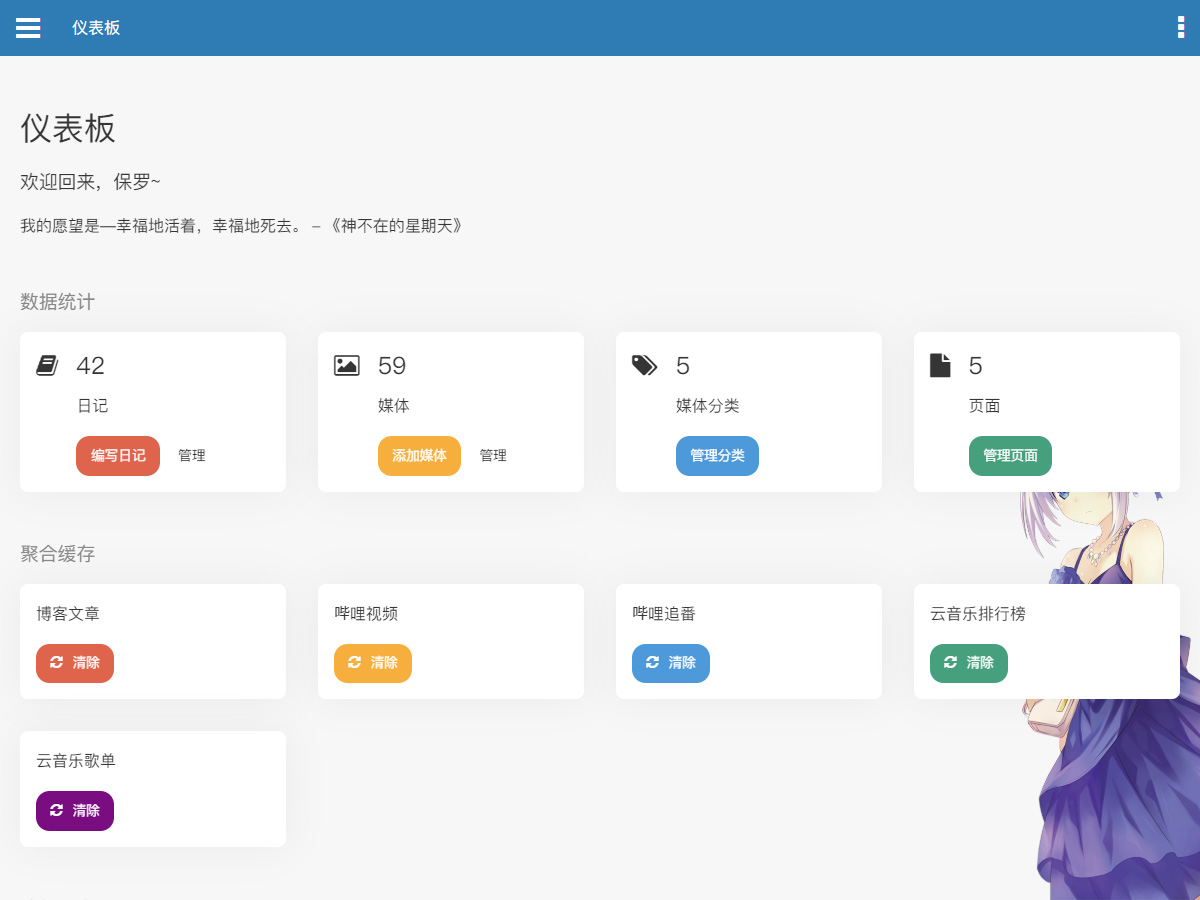# 2020-02-25（周二）

``````let [a, b, c] = [5, 1, 2];
// 等同于 let a = 5, b = 1, c = 2;

let arr = [4, 5];
let [x, y, z] = arr;  // 等同于 let x = 4, y = 5, z = undefined
let [a, b, c] = [1, [1, 2], {id: 1}];  // 等同于 let a = 1,  b = [1, 2], c = {id: 1}
let [a, [b], d] = [1, [2, 3], 4];  // 等同于 let a = 1, b = 2, d = 4;

let arr2 = [100, 200];
let [x = 1, y = 2] = arr2;
// 等同于 let x = 100, 200，因为有内容，如果不用默认参数，就是
// let x = arr2 || 1, y = arr2 || 2;

let [x = 1, y = 2] = ;  // 等同于 let x = 6, y = 2
let [x = 10, y = 20] = [];  // 等同于 let x = 10, y = 20
let [x, y = 100] = ;  // 等同于 let x = 10, y = 100

// 以下两种情况只有 undefined 会变成 1
let [x = 1] = [null]
let [x = 1] = [undefined]``````

``````let obj = {
name: "Paul",
age: 19,
id: 1
}
let {name, age} = obj;  // 等同于 let name = obj.name, age = obj.age
let {name:n, age:a} = obj;  // 等同于 let n = obj.name, a = obj.age

let {a} = {x: 1, y: 2};  // 无法匹配
let {x:a} = {x: 1, y: 2};  // 等同于 let a = 1，a 作为变量，x 作为匹配模式

let {x = 1, y = 10} = {x:undefined};  // 等同于 let x = 1, y = 10
let {x:y = 5} = {x: 10};  // 等同于 let y = 10``````

``````function test([x = 1, y = 2]) {
return x + y;
}
test();

function test2(x, y) {
x = x || 1;
y = y || 2;
return x + y;
}
test2(10);

function test3({x = 1, y = 2}){
return x + y;
}
test3({x: 10})``````

# 2020-02-21（周五）# 2020-02-14（周五）

``````// 获取输入框
static getInput(name){
return document.getElementsByName(name);
}

static getInput2 = (name) => document.getElementsByName(name);

static getInput3 = (name) => {
return document.getElementsByName(name);
}``````

``````function push(array, ...items) {
items.forEach(function(item) {
array.push(item);
console.log(item);
});
}

var a = [];
push(a, 1, 2, 3)``````

# 2020-02-13（周四）

``````\$uid = \$bgm_user ? \$bgm_user : \$mode === "bili" ? 124512959 : 433599;
\$uid = \$bgm_user ? \$bgm_user : (\$mode === "bili" ? 124512959 : 433599);``````

# 2020-02-12（周三）Emmm，这里是歌词君